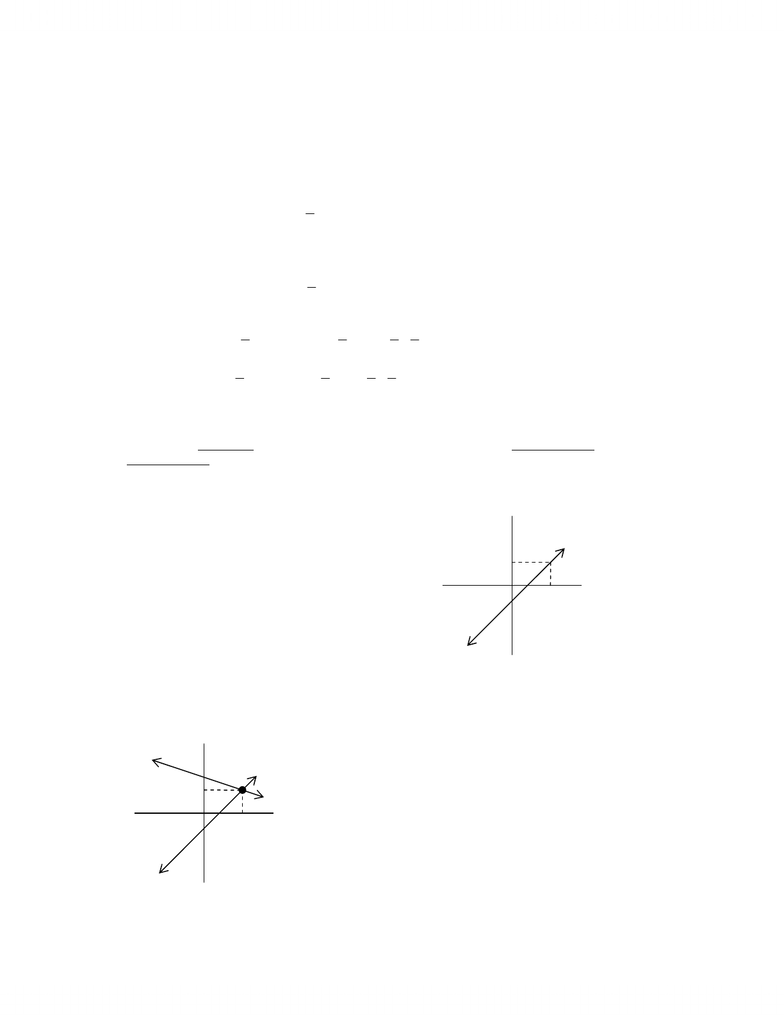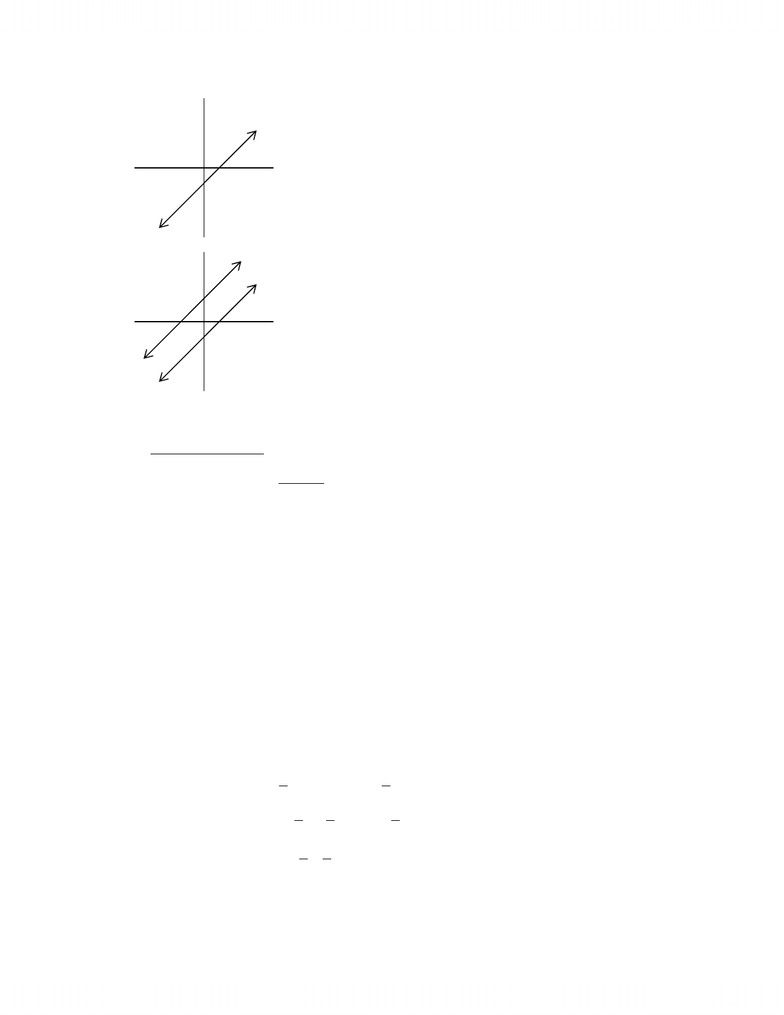# Summary notes 1

151 views37 pages
School
Department
Course
ProfessorMAT223H1a.doc
Page 1 of 38
Lecture #1 Tuesday, January 6, 2004
1.1 SOLUTIONS AND ELEMENTARY OPERATIONS
Example
Create a diet from fish and meal that contains 193g of proteins and 83g of carbohydrate. We know that fish
contains 70% protein and 10% carbohydrate, and meal contains 30% protein and 60% carbohydrate.
Assume that the diet contains xg of fish and yg of meal, we obtain
=+
=+
836.01.0
1933.07.0
yx
yx .
Definition
A linear equation is an equation of the form bxaxann =++ ...
11 where:
x1,, xn are variables;
a1,, an are real numbers called coefficients;
b is the constant term.
Examples
1) cbyax=+ .
2) 023 321 =+ xxx .
3) 12 2
2
1=+ xx not a linear equation.
Definition
A finite collection of linear equations in the variables x1,, xn is called a system of linear equations.
Examples
1)
=
=
3
1
2
1
x
x.
2)
=
=+
03
1442
21
21
xx
xx
Definition
Given a linear equation bxaxann =++ ...
11 , a sequence of n real numbers s1,, sn is called a solution to the
linear equation if bsasann =++ ...
11 . Similarly, this also applies to a given system of linear equations.
Example
Given
( )
=
=
3
1
2
1
1x
x
S and
( )
=
=+
03
1442
21
21
2xx
xx
S, what is the solution to (S1) and (S2)?
(1, 3) is a solution of (S1).
(1, 3) is also a solution of (S2) because
(
)
(
)
143412 =+ and
(
)
(
)
0313 =.
www.notesolution.com
Unlock document

This preview shows pages 1-3 of the document.
Unlock all 37 pages and 3 million more documents.MAT223H1a.doc
Page 2 of 38
Example
( )
=+
=+
2
1
3yx
yx
S has no solution.
Example
Prove that for any s, t in R,
=
=
=
+=
ss
ts
ss
tss
2
2
1
2
3
4
3
2
1
is a solution to the system
=+++
=+
2
13
4321
4321
xxxx
xxxx .
13
2
1
2
3
2
2
1
3
2
3
34321 =+++=
++=+ttsssststsssss .
22
2
1
2
3
2
2
1
2
3
4321 =++++=++++=+++ ttsssststsssss .
Definition
s, t are called parameters. s1,, s4 described this way is said to be given in parametric form and is called the
general solution of the system.
Remarks
When only 2 variables are involved, solution to
systems of linear equations can be described
geometrically because a linear equation
cbyaxL=+: is a straight line if a, b are not
both 0.
(
)
21 ,ssP is in L if it is a solution of
cbyax=+ .
If there are two linear equations, cbyaxL=+:
1 and feydxL=+:
2, then the solution to the system
( )
=+
=+
feydx
cbyax
S is the intersection of L1 and L2.
The solution of (S) is
(
)
21 ,ss where
(
)
2121 ,LLssP=.
www.notesolution.com
Unlock document

This preview shows pages 1-3 of the document.
Unlock all 37 pages and 3 million more documents.MAT223H1a.doc
Page 3 of 38
The solution of (S) are given by the
(
)
21 ,ss
such that cbsas=+ 21 (and this implies that
fesds=+ 21 ).
(S) has no solution.
Definition
The elementary operations are:
1) Interchange 2 equations.
2) Multiply one equation by a non-zero number.
3) Add a multiple of one equation to a different equation.
Definition
Two systems of linear equations are said to be equivalent iff the solutions of the systems are the same.
Theorem
Suppose that an elementary operation is performed on a system of linear equations, then the resulting system
is equivalent to the original one.
Example
Solve:
=
=+
43
12
yx
yx .
=
=
==
=
=
=+
=
=
+=
=
=+
=
=
=+
=
=+
7
1
7
9
7
2
7
9
12
7
9
12
97
97
12
2826
12
43
12
12
21
212
1
22
1
2
1
y
x
y
x
RRyx
RRx
RRRx
Ryx
RRyx
Ryx
Ryx
Ryx
The solution of the system is
7
1
,
7
9.
L
1
www.notesolution.com
Unlock document

This preview shows pages 1-3 of the document.
Unlock all 37 pages and 3 million more documents.

## Get access